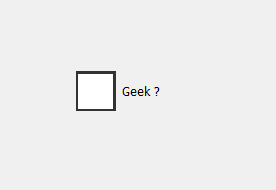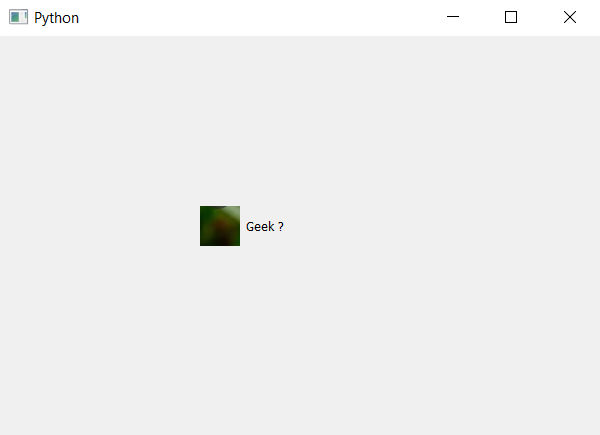# PyQt5 – Image Indicator of Check Box

• Last Updated : 22 Apr, 2020

Check box is basically divided into two components one is the indicator which is on the left side by default and other is the check box itself i.e the label part which has text on it. In this article we will see how to set image to a indicator.

Below is the representation of normal indicator of check box vs the check box with image on indicator.In order to set image to a indicator we have to change the style sheet of the indicator of the check box. Below is the style sheet code which is used with the check box object.

```QCheckBox::indicator
{
background-image :url(image.png);
}
```

Below is the implementation.

 `# importing libraries``from` `PyQt5.QtWidgets ``import` `*` `from` `PyQt5 ``import` `QtCore, QtGui``from` `PyQt5.QtGui ``import` `*` `from` `PyQt5.QtCore ``import` `*` `import` `sys`` ` ` ` `class` `Window(QMainWindow):`` ` `    ``def` `__init__(``self``):``        ``super``().__init__()`` ` `        ``# setting title``        ``self``.setWindowTitle(``"Python "``)`` ` `        ``# setting geometry``        ``self``.setGeometry(``100``, ``100``, ``600``, ``400``)`` ` `        ``# calling method``        ``self``.UiComponents()`` ` `        ``# showing all the widgets``        ``self``.show()`` ` `    ``# method for widgets``    ``def` `UiComponents(``self``):`` ` `        ``# creating the check-box``        ``checkbox ``=` `QCheckBox(``'Geek ?'``, ``self``)`` ` `        ``# setting geometry of check box``        ``checkbox.setGeometry(``200``, ``150``, ``100``, ``80``)`` ` `        ``# setting stylesheet``        ``# adding background image to indicator of check box ``        ``# and changing with and height of indicator``        ``checkbox.setStyleSheet(``"QCheckBox::indicator"``                               ``"{"``                               ``"background-image : url(image.png);"``                               ``"width :40px;"``                               ``"height : 40px;"``                               ``"}"``)`` ` ` ` ` ` `# create pyqt5 app``App ``=` `QApplication(sys.argv)`` ` `# create the instance of our Window``window ``=` `Window()`` ` `# start the app``sys.exit(App.``exec``())`

Output :My Personal Notes arrow_drop_up